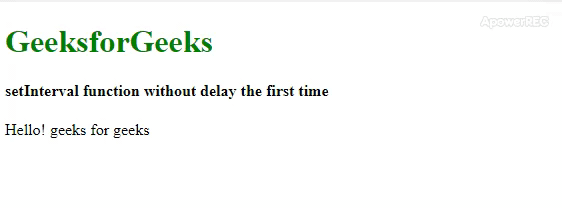# How to trigger setInterval loop immediately using JavaScript ?

This article will show some simple ways in which the setInterval() method can be made to execute the function without delay. There are many procedures to do so, below all the procedures are described with the example.

Note: In this article setInterval() method will start immediately for the 1st time run.

Below examples illustrate the above approach:

Example 1: Here, the setInterval() method is returned in gfg() function. The gfg() function trigger itself for subsequent calls using setTimeout() instead. Multiply the output each time in this method.

 ` ` `<``html``> ` ` `  `<``head``> ` `    ``<``title``> ` `        ``Start setInterval loop immediately ` `    `` ` ` ` ` `  `<``body``> ` `    ``<``h1` `style``=``"color: green;"``> ` `        ``GeeksforGeeks ` `    `` ` `     `  `    ``<``h4``> ` `        ``Start setInterval loop immediately ` `    `` ` `     `  `    ``<``script``> ` `        ``function gfg() { ` `            ``document.write("Hello! geeks" ` `                        ``+ " for geeks<``br``>"); ` `            ``return setInterval(gfg, 3000); ` `        ``} ` ` `  `        ``// Function call ` `        ``gfg(); ` `    `` ` ` ` ` `  ` `

Output:Example 2: This can be implemented using immediately invoked function expressions (IIFE) . It defines the function gfg() and calls in one go. Multiply the output each time in this method.

 ` ` `<``html``> ` `  `  `<``head``> ` `    ``<``title``> ` `        ``Start setInterval loop immediately ` `    `` ` ` ` `  `  `<``body``> ` `    ``<``h1` `style``=``"color: green;"``> ` `        ``GeeksforGeeks ` `    `` ` `     `  `    ``<``h4``> ` `        ``Start setInterval loop immediately ` `    `` ` `     `  `    ``<``script``> ` `  `  `        ``// Using IIFE ` `        ``(function gfg() { ` `            ``document.write("Hello! geeks" ` `                    ``+ " for geeks<``br``>"); ` `            ``return setInterval(gfg, 3000); ` `        ``})(); ` `    `` ` ` ` `  `  ` `

Output:Example 3: The simplest way is calling the gfg() first and then starting the setInterval’s cycle of execution.

 ` ` `<``html``> ` `  `  `<``head``> ` `    ``<``title``> ` `        ``Start setInterval loop immediately ` `    `` ` ` ` `  `  `<``body``> ` `    ``<``h1` `style``=``"color: green;"``> ` `        ``GeeksforGeeks ` `    `` ` `     `  `    ``<``h4``> ` `        ``Start setInterval loop immediately ` `    `` ` `     `  `    ``<``script``> ` `        ``function gfg() { ` `            ``document.write("Hello! geeks" ` `                    ``+ " for geeks<``br``>") ` `        ``} ` `  `  `        ``gfg(); ` `        ``setInterval(gfg, 3000); ` `    `` ` ` ` `  `  ` `

Output:In all the above code, the first time “Hello! geeks for geeks” statement will be displayed immediately followed by the second and third and so on with a time interval of 3 seconds. The first time gfg() will be called immediately after running the code.

Note: All the above examples can be tested by typing them within the script tag of HTML or directly into the browser’s console.My Personal Notes arrow_drop_upCheck out this Author's contributed articles.

If you like GeeksforGeeks and would like to contribute, you can also write an article using contribute.geeksforgeeks.org or mail your article to contribute@geeksforgeeks.org. See your article appearing on the GeeksforGeeks main page and help other Geeks.

Please Improve this article if you find anything incorrect by clicking on the "Improve Article" button below.